# Steffensen interpolation formula

(diff) ← Older revision | Latest revision (diff) | Newer revision → (diff)

A form of notation of the interpolation polynomial obtained from the Stirling interpolation formula by means of the nodes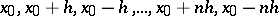at a point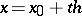: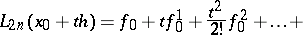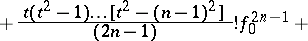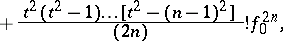using the relations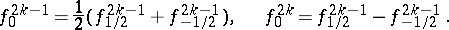After collecting similar terms, the Steffensen interpolation formula can be written in the form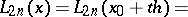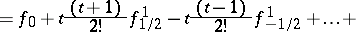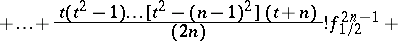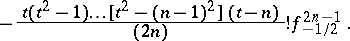How to Cite This Entry:
Steffensen interpolation formula. Encyclopedia of Mathematics. URL: http://encyclopediaofmath.org/index.php?title=Steffensen_interpolation_formula&oldid=13445
This article was adapted from an original article by M.K. Samarin (originator), which appeared in Encyclopedia of Mathematics - ISBN 1402006098. See original article